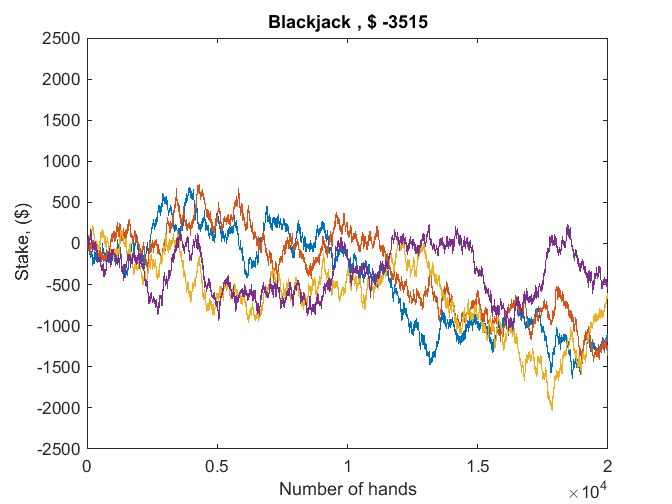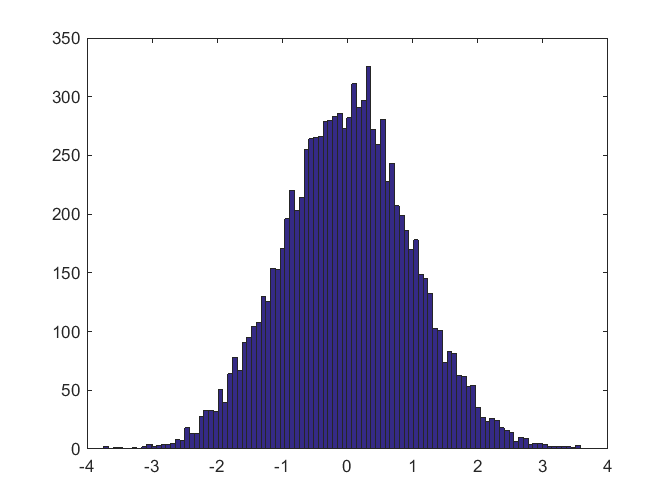# How Far Apart Are Two Random Points in a Hypercube?3

Two days ago I wrote about random points in a square. At the last minute I added the paragraph asking about the generalization to random points in a cube. I have to admit that I didn't check the Web to see what was known about the question.... 続きを読む >>

# Parallel Random Number GeneratorsThis is the second of a multi-part series about the MATLAB random number generators. If you ask for help rng, you will get lots of information, including the fact that there are three modern generators.... 続きを読む >>

# Random Number Generators, Mersenne TwisterThis is the first of a multi-part series about the MATLAB random number generators.... 続きを読む >>